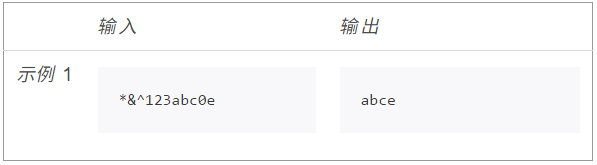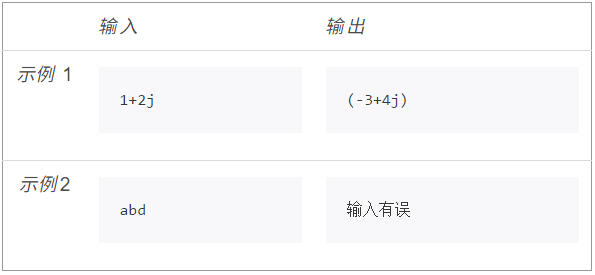# 《Python语言程序设计》【第8章】测试笔记

1、
os库是Python重要的标准库之一，提供了几百个函数功能，覆盖与操作系统、文件操作等相关的众多功能。os库适合所有操作系统。

2、

3、

4、

5、

6、

7、

1、

## 描述def getInput():
s = input()
return s

def printWord(s):
ss = ""
for c in s:
if 'a' <= c <= 'z':
ss += c
print(ss)

def main():
s = getInput()
printWord(s)

main()

【参考答案】

alpha = []
for i in range(26):
alpha.append(chr(ord('a') + i))
alpha.append(chr(ord('A') + i))
s = input()
for c in s:
if c in alpha:
print(c, end="")

2、

## 描述

（1）无论用户输入何种内容，程序无错误；‪‬‪‬‪‬‪‬‪‬‮‬‪‬‫‬‪‬‪‬‪‬‪‬‪‬‮‬‭‬‪‬‪‬‪‬‪‬‪‬‪‬‮‬‪‬‭‬‪‬‪‬‪‬‪‬‪‬‮‬‪‬‮‬‪‬‪‬‪‬‪‬‪‬‮‬‪‬‪‬‪‬‪‬‪‬‪‬‪‬‮‬‫‬‮‬

（2）如果输入有误，请输出"输入有误"。def getInput():
num = input()
return num

def printNum(n):
try:
if complex(n) == complex(eval(n)):
num = pow(eval(n), 2)
print(num)
else:
print("输入有误")
except:
print("输入有误")

def main():
num = getInput()
printNum(num)

main()

s = input()
try:
if complex(s) == complex(eval(s)):
print(eval(s)**2)
except:
print("输入有误")

complex()和complex(eval())之间的比较将能够排除非数字类型的输入。

【注】不能直接使用eval()，否则，用户可以通过输入表达式（如100**2）输入数字，与要求不同（在实际应用中带来安全隐患）。

06-061万+03-06244
08-034万+
04-20241
04-081365
08-06390
06-119333
04-18648
02-28905
03-17899